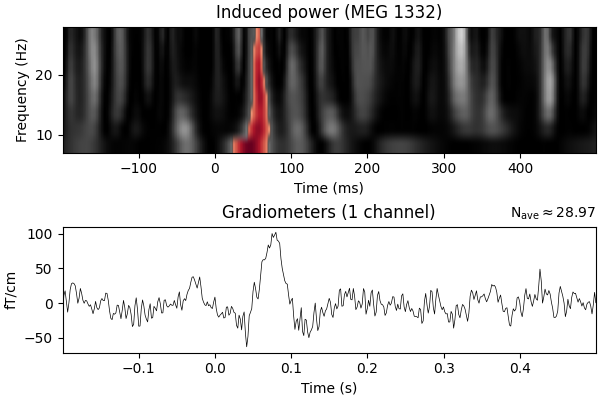# Non-parametric between conditions cluster statistic on single trial power#

This script shows how to compare clusters in time-frequency power estimates between conditions. It uses a non-parametric statistical procedure based on permutations and cluster level statistics.

The procedure consists of:

• extracting epochs for 2 conditions

• compute single trial power estimates

• baseline line correct the power estimates (power ratios)

• compute stats to see if the power estimates are significantly different between conditions.

```# Authors: Alexandre Gramfort <alexandre.gramfort@inria.fr>
#
```
```import matplotlib.pyplot as plt
import numpy as np

import mne
from mne.datasets import sample
from mne.stats import permutation_cluster_test
from mne.time_frequency import tfr_morlet

print(__doc__)
```

Set parameters

```data_path = sample.data_path()
meg_path = data_path / "MEG" / "sample"
raw_fname = meg_path / "sample_audvis_raw.fif"
event_fname = meg_path / "sample_audvis_raw-eve.fif"
tmin, tmax = -0.2, 0.5

# Setup for reading the raw data

include = []

picks = mne.pick_types(
raw.info,
eeg=False,
eog=True,
stim=False,
include=include,
)

ch_name = "MEG 1332"  # restrict example to one channel

event_id = 1
epochs_condition_1 = mne.Epochs(
raw,
events,
event_id,
tmin,
tmax,
picks=picks,
baseline=(None, 0),
reject=reject,
)
epochs_condition_1.pick([ch_name])

event_id = 2
epochs_condition_2 = mne.Epochs(
raw,
events,
event_id,
tmin,
tmax,
picks=picks,
baseline=(None, 0),
reject=reject,
)
epochs_condition_2.pick([ch_name])
```
```Opening raw data file /home/circleci/mne_data/MNE-sample-data/MEG/sample/sample_audvis_raw.fif...
Read a total of 3 projection items:
PCA-v1 (1 x 102)  idle
PCA-v2 (1 x 102)  idle
PCA-v3 (1 x 102)  idle
Range : 25800 ... 192599 =     42.956 ...   320.670 secs
72 matching events found
Setting baseline interval to [-0.19979521315838786, 0.0] s
Applying baseline correction (mode: mean)
3 projection items activated
Rejecting  epoch based on EOG : ['EOG 061']
Rejecting  epoch based on EOG : ['EOG 061']
Rejecting  epoch based on EOG : ['EOG 061']
Rejecting  epoch based on EOG : ['EOG 061']
Rejecting  epoch based on EOG : ['EOG 061']
Rejecting  epoch based on EOG : ['EOG 061']
Rejecting  epoch based on EOG : ['EOG 061']
Rejecting  epoch based on EOG : ['EOG 061']
Rejecting  epoch based on EOG : ['EOG 061']
Rejecting  epoch based on EOG : ['EOG 061']
Rejecting  epoch based on EOG : ['EOG 061']
Rejecting  epoch based on EOG : ['EOG 061']
Rejecting  epoch based on EOG : ['EOG 061']
Rejecting  epoch based on EOG : ['EOG 061']
Rejecting  epoch based on EOG : ['EOG 061']
Rejecting  epoch based on EOG : ['EOG 061']
Removing projector <Projection | PCA-v1, active : True, n_channels : 102>
Removing projector <Projection | PCA-v2, active : True, n_channels : 102>
Removing projector <Projection | PCA-v3, active : True, n_channels : 102>
73 matching events found
Setting baseline interval to [-0.19979521315838786, 0.0] s
Applying baseline correction (mode: mean)
3 projection items activated
Rejecting  epoch based on EOG : ['EOG 061']
Rejecting  epoch based on EOG : ['EOG 061']
Rejecting  epoch based on EOG : ['EOG 061']
Rejecting  epoch based on EOG : ['EOG 061']
Rejecting  epoch based on EOG : ['EOG 061']
Rejecting  epoch based on EOG : ['EOG 061']
Rejecting  epoch based on EOG : ['EOG 061']
Rejecting  epoch based on EOG : ['EOG 061']
Rejecting  epoch based on EOG : ['EOG 061']
Rejecting  epoch based on EOG : ['EOG 061']
Rejecting  epoch based on EOG : ['EOG 061']
Rejecting  epoch based on EOG : ['EOG 061']
Rejecting  epoch based on EOG : ['EOG 061']
Removing projector <Projection | PCA-v1, active : True, n_channels : 102>
Removing projector <Projection | PCA-v2, active : True, n_channels : 102>
Removing projector <Projection | PCA-v3, active : True, n_channels : 102>
```
Number of events 60 2: 60 -0.200 – 0.499 s -0.200 – 0.000 s

Factor to downsample the temporal dimension of the TFR computed by tfr_morlet. Decimation occurs after frequency decomposition and can be used to reduce memory usage (and possibly comptuational time of downstream operations such as nonparametric statistics) if you don’t need high spectrotemporal resolution.

```decim = 2
freqs = np.arange(7, 30, 3)  # define frequencies of interest
n_cycles = 1.5

tfr_epochs_1 = tfr_morlet(
epochs_condition_1,
freqs,
n_cycles=n_cycles,
decim=decim,
return_itc=False,
average=False,
)

tfr_epochs_2 = tfr_morlet(
epochs_condition_2,
freqs,
n_cycles=n_cycles,
decim=decim,
return_itc=False,
average=False,
)

tfr_epochs_1.apply_baseline(mode="ratio", baseline=(None, 0))
tfr_epochs_2.apply_baseline(mode="ratio", baseline=(None, 0))

epochs_power_1 = tfr_epochs_1.data[:, 0, :, :]  # only 1 channel as 3D matrix
epochs_power_2 = tfr_epochs_2.data[:, 0, :, :]  # only 1 channel as 3D matrix
```
```Not setting metadata
Applying baseline correction (mode: ratio)
Applying baseline correction (mode: ratio)
```

## Compute statistic#

```threshold = 6.0
F_obs, clusters, cluster_p_values, H0 = permutation_cluster_test(
[epochs_power_1, epochs_power_2],
n_permutations=100,
threshold=threshold,
tail=0,
seed=np.random.default_rng(seed=8675309),
)
```
```stat_fun(H1): min=0.000000 max=13.566824
Running initial clustering …
Found 6 clusters

0%|          | Permuting : 0/99 [00:00<?,       ?it/s]
30%|███       | Permuting : 30/99 [00:00<00:00,  873.81it/s]
63%|██████▎   | Permuting : 62/99 [00:00<00:00,  909.35it/s]
95%|█████████▍| Permuting : 94/99 [00:00<00:00,  922.49it/s]
100%|██████████| Permuting : 99/99 [00:00<00:00,  922.73it/s]
```

## View time-frequency plots#

```times = 1e3 * epochs_condition_1.times  # change unit to ms

fig, (ax, ax2) = plt.subplots(2, 1, figsize=(6, 4), layout="constrained")

# Compute the difference in evoked to determine which was greater since
# we used a 1-way ANOVA which tested for a difference in population means
evoked_power_1 = epochs_power_1.mean(axis=0)
evoked_power_2 = epochs_power_2.mean(axis=0)
evoked_power_contrast = evoked_power_1 - evoked_power_2
signs = np.sign(evoked_power_contrast)

# Create new stats image with only significant clusters
F_obs_plot = np.nan * np.ones_like(F_obs)
for c, p_val in zip(clusters, cluster_p_values):
if p_val <= 0.05:
F_obs_plot[c] = F_obs[c] * signs[c]

ax.imshow(
F_obs,
extent=[times, times[-1], freqs, freqs[-1]],
aspect="auto",
origin="lower",
cmap="gray",
)
max_F = np.nanmax(abs(F_obs_plot))
ax.imshow(
F_obs_plot,
extent=[times, times[-1], freqs, freqs[-1]],
aspect="auto",
origin="lower",
cmap="RdBu_r",
vmin=-max_F,
vmax=max_F,
)

ax.set_xlabel("Time (ms)")
ax.set_ylabel("Frequency (Hz)")
ax.set_title(f"Induced power ({ch_name})")

# plot evoked
evoked_condition_1 = epochs_condition_1.average()
evoked_condition_2 = epochs_condition_2.average()
evoked_contrast = mne.combine_evoked(
[evoked_condition_1, evoked_condition_2], weights=[1, -1]
)
evoked_contrast.plot(axes=ax2, time_unit="s")
``````Need more than one channel to make topography for grad. Disabling interactivity.
```

Total running time of the script: (0 minutes 6.000 seconds)

Estimated memory usage: 10 MB

Gallery generated by Sphinx-Gallery